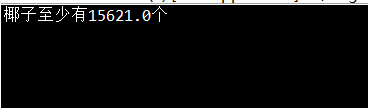算法学习——递推之水手分椰子

算法思路

1. 这里需要注意的是，没有初始条件，求最初最少摘了多少个椰子

2. y[i] 代表第i个水手偷藏的椰子

由题目可以得到 个迭代方程 n/5 = (n-n/5-1)/5

这里等式左边是第1个水手所藏的椰子数，右边则是下一个水手所藏的椰子数

化简可得递推公式y[i+1]=(4y[i]-1)/5 从前往后推

3. 当每次递推的所藏椰子数为正整数，则满足条件，这里通过使用floor函数可以判定一个数是否为整数

算法实现

int i =1;
double k,y,x;
k=1.0;
y=k;
while(i<=5){
i++;
y = (4*y-1)/5;
if(y!=Math.floor(y)){
k++;
y=k;
i=1;
}
}
x=5*k+1;
System.out.println("椰子至少有"+x+"个");

结果posted @ 2018-11-06 16:46  Stars-one  阅读(1391)  评论(0编辑  收藏  举报